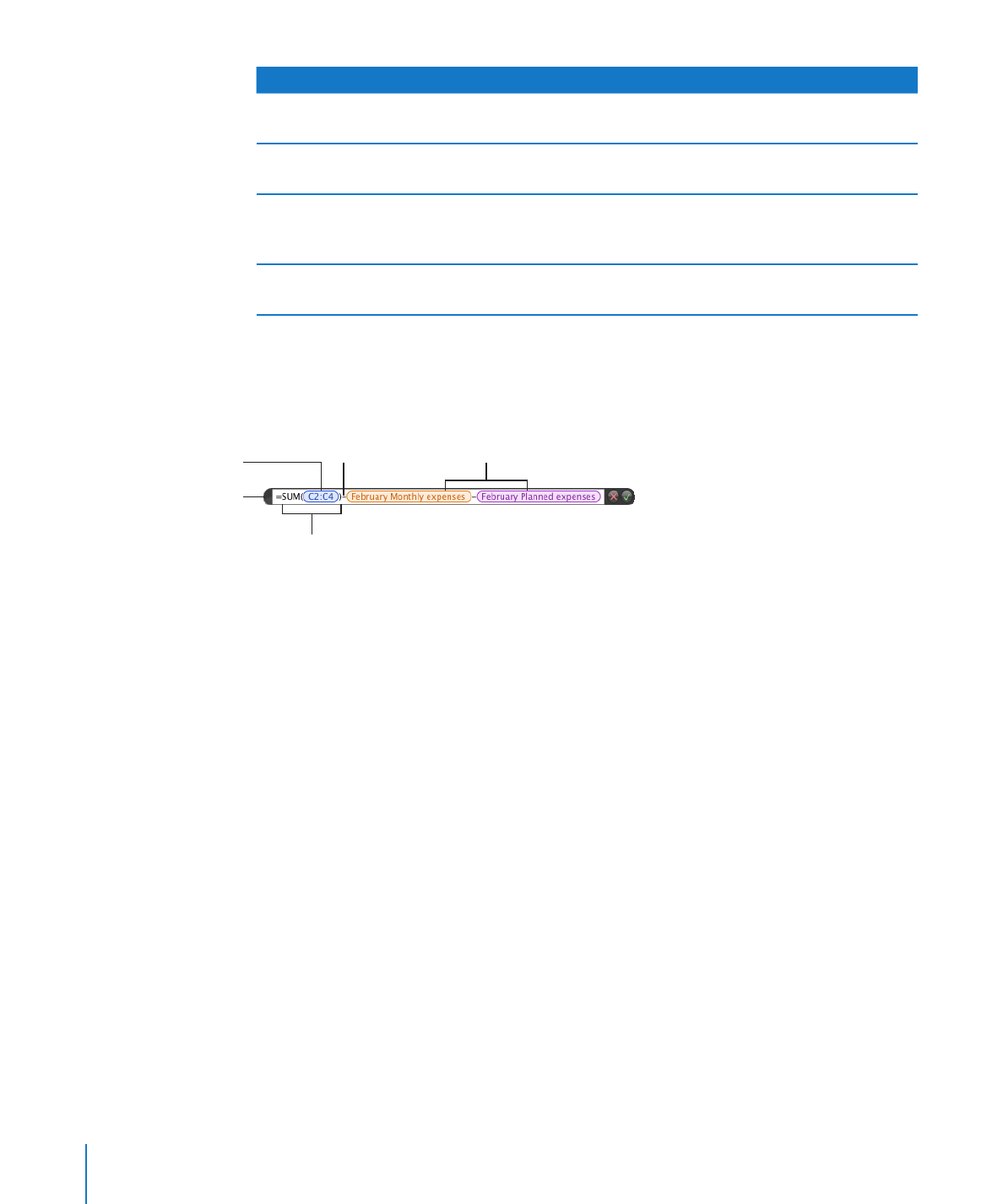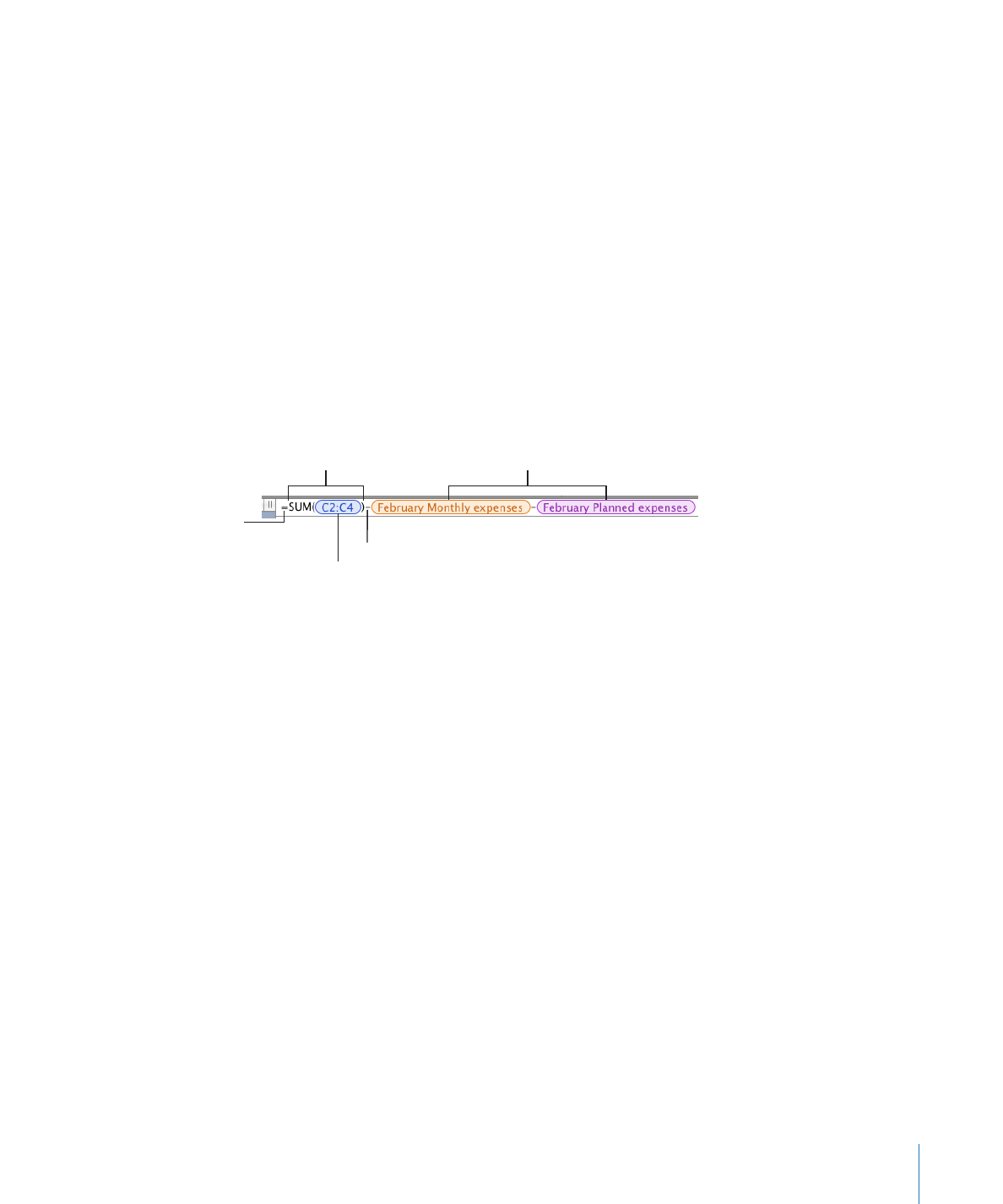## Numbers - Adding and Editing Formulas Using the Formula EditorAdding and Editing Formulas Using the Formula Editor

The Formula Editor has a text field that holds your formula. As you add cell references,
operators, functions, or constants to a formula, they look like this in the Formula Editor.

All formulas must begin

with the equal sign.

The Sum function.

References to cells
using their names.

A reference to a

range of three cells.

The Subtraction
operator.

Here are ways to work with the Formula Editor:
To open the Formula Editor, do one of the following:

m

Select a table cell and then type the equal sign (=).

Â

Double-click a table cell that contains a formula.

Â

Select a table cell, click Function in the toolbar, and then choose Formula Editor

Â

Select a table cell and then choose Insert > Function > Formula Editor.

Â

Select a cell that contains a formula, and then press Option-Return.

Â

The Formula Editor opens over the selected cell, but you can move it.
To move the Formula Editor, hold the pointer over the left side of the Formula Editor

m

until it changes into a hand, and then drag.
To build your formula, do the following:

m

To add an operator or a constant to the text field, place the insertion point and

Â

type. You can use the arrow keys to move the insertion point around in the text
field. See “Using Operators in Formulas” on page 130 to learn about operators you
can use. When your formula requires an operator and you haven’t added one, the
+ operator is inserted automatically. Select the + operator and type a different
operator if needed.

122

Chapter 6

Using Formulas in TablesChapter 6

Using Formulas in Tables

123

To add cell references to the text field, place the insertion point and follow the

Â

instructions in “Referring to Cells in Formulas” on page 126.
To add functions to the text field, place the insertion point and follow the

Â

instructions in “Adding Functions to Formulas” on page 124.

To remove an element from the text field, select the element and press Delete.

m

To save changes, press Return, press Enter, or click the Accept button in the Formula

m

Editor. You can also click outside the table.
To close the Formula Editor and not save any changes you made, press Esc or click the
Cancel button in the Formula Editor.